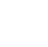# Java Branching and Looping Excerise

## Problem Statement-1:

Write a Java program that reads an integer and check whether it is negative, zero, or positive

Test Data:

Input a number: 7

Expected Output :

Input a number: 7

Number is positive

Solution:

import java.util.Scanner;
public class Exercise1{
public static void main(String[] args)
{
Scanner in = new Scanner(System.in);
System.out.print("Input a number: ");
int n = in.nextInt();

if (n > 0)
{
System.out.println("Number is positive");
}
else if (n < 0)
{
System.out.println("Number is negative");
}
else
{
System.out.println("Number is zero");
}
}
}

/*OUTPUT:
Input a number: 7
Number is positive */

Explanation:

The class Exercise1 contains the field int n, the Scanner class gets the value from the user. If the n value is greater than 0, it executes if block. When n is less than 0 it executes else if block. If none of the conditions is satisfied it executes the else block. The Scanner is a class in java. util package used for obtaining the input of the primitive types like int, double, etc., and strings.

## Problem Statement-2:

Write a java program that prints the even numbers.

Test Data:

Enter the limit: 20

Expected Output :

Enter the limit: 20

List of even numbers: 2 4 6 8 10  12  14  16  18  20

Solution:

import java.util.Scanner;
public class Exercise2 {
public static void main(String[] args) {
int number, i;
Scanner sc = new Scanner(System.in);
System.out.print("Enter the limit: ");
number = sc.nextInt();
i = 2;
System.out.print("List of even numbers: ");
//the while loop executes until the condition become false
while (i <= number) {
//prints the even number
System.out.print(i + " ");
//increments the variable i by 2
i = i + 2;
}
}
}

/*OUTPUT:
Enter the limit: 20
List of even numbers: 2 4 6 8 10  12  14  16  18  20 */

Explanation:

The class Exercise2 contains fields such as int number, i. The Scanner class is used to get values from users during run time. The value 2 is assigned to the variable i. When while(i <= number) is satisfied the loop iterates and increments until the condition fails.  The Scanner is a class in java. util package used for obtaining the input of the primitive types like int, double, etc., and strings.